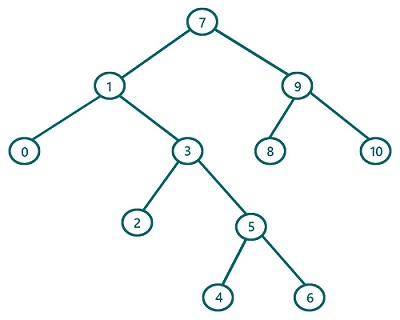# Reverse Preorder Traversal in Binary Tree (with recursion) in C, C++

In this article, we are going to find what reverse preorder traversal of a Binary Tree is and how to implement reverse preorder traversal using recursion? We have provided the implementation both in C & C++.
Submitted by Radib Kar, on July 24, 2020

If we classify tree traversals, preorder traversal is one of traversal which is based on depth-first search traversal.  Reverse preorder traversal is a modified version of preorder traversal sometimes needed for solving tree problems. The basic concept for reverse preorder traversal remains exactly same as of the preorder traversal, except the subtree traverse order (which one will be traversed first b/w right and left). In normal preorder traversal we saw that

1. First, traverse the root
2. Then, traverse the left subtree
3. Finally, traverse the right subtree

But in reverse preorder traversal instead of traversing the left subtree first, we traverse the right first and then the left subtree. So the updated rules for reverse inorder traversal is:

1. First, traverse the root
2. Then traverse the right subtree
3. Finally, traverse the left subtree

Of course, while traversing the subtrees we will follow the same order

So let's traverse the below tree using reveerse preorder traversal.For the above tree, the root is: 7

So first print root -> 7

Traversal till now: 7

Then Traverse the right subtree (Subtree rooted by 9)

Now to traverse the subtree rooted by 9, it again follows the same set of rules

So First print 9

Traversal till now: 7 9

Then traverse the right subtree of this (rooted by 10)

Since it's a leaf node we can print it as there is no more right subtree. Also, 10 has no left subtree, so this subtree rooted by 10 is traversed totally.

Traversal till now: 7 9 10

Since the right subtree of the subtree rooted 9 is traversed, now rest is traversing the left subtree. So it will traverse 8 then

Traversal till now: 7 9 10 8

At this stage we are done with processing the traversal of right subtree of the original tree root(7). Rest is about processing the left subtree.So process the left siubtree rooted by 1 in the same way.

After processing left subtree, traversal of the tree rooted by 1 in the same way we will have traversal: 7 9 10 8 1 3 5 6 4 2 0 which is our final reverse preorder traversal result.

The pseudocode would be:

```void reverse_preorder (TreeNode root){
if(root is NULL)
return

//traverse current node
print(root)
// recursively traverse the right subtree
reverse_preorder (right subtree of the root)
//recursively traverse the left subtree
reverse_preorder (left subtree of the root)
}
```

C Implementation:

```#include <stdio.h>
#include <stdlib.h>

struct tree {
int val;
struct tree* left;
struct tree* right;
};

typedef struct tree TreeNode;

TreeNode* newTree(int data)
{
// Allocate memory for new node
TreeNode* root = (TreeNode*)malloc(sizeof(TreeNode));

// Assign data to this node val
root->val = data;

// Initialize left and right children as NULL
root->left = NULL;
root->right = NULL;
return (root);
}

void reverse_preorder(TreeNode* root)
{
//base case
if (root == NULL)
return;

//First traverse current node
printf("%d ", root->val);

//secondly traverse right sub tree
reverse_preorder(root->right);

// Finally traverse left sub tree
reverse_preorder(root->left);
}

int main()
{
//building the tree
TreeNode* t = newTree(7);

t->left = newTree(1);
t->left->left = newTree(0);
t->left->right = newTree(3);
t->left->right->left = newTree(2);
t->left->right->right = newTree(5);
t->left->right->right->left = newTree(4);
t->left->right->right->right = newTree(6);
t->right = newTree(9);
t->right->left = newTree(8);
t->right->right = newTree(10);

printf("Reverse preorder traversal of the above tree is:\n");
reverse_preorder(t);

return 0;
}
```

Output:

```Reverse preorder traversal of the above tree is:
7 9 10 8 1 3 5 6 4 2 0
```

C++ implementation:

```#include <bits/stdc++.h>
using namespace std;

class TreeNode { // tree node is defined
public:
int val;
TreeNode* left;
TreeNode* right;
TreeNode(int data)
{
val = data;
left = NULL;
right = NULL;
}
};

void reverse_preorder(TreeNode* root)
{
//base case
if (root == NULL)
return;
//First traverse current node
printf("%d ", root->val);
//secondly traverse right sub tree
reverse_preorder(root->right);
// Finally traverse left sub tree
reverse_preorder(root->left);
}

int main()
{
//building the tree
TreeNode* t = new TreeNode(7);

t->left = new TreeNode(1);
t->left->left = new TreeNode(0);
t->left->right = new TreeNode(3);
t->left->right->left = new TreeNode(2);
t->left->right->right = new TreeNode(5);
t->left->right->right->left = new TreeNode(4);
t->left->right->right->right = new TreeNode(6);
t->right = new TreeNode(9);
t->right->left = new TreeNode(8);
t->right->right = new TreeNode(10);

printf("Reverse preorder traversal of the above tree is:\n");
reverse_preorder(t);

return 0;
}
```

Output:

```Reverse preorder traversal of the above tree is:
7 9 10 8 1 3 5 6 4 2 0
```